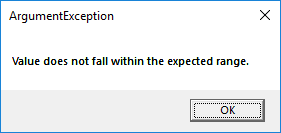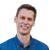# Value does not fall within expected range

1326
2
11-28-2018 02:06 PMNew Contributor II

I use Arcgis runtime for net 100.2 and create C#/WPF application.

I developed custom circle (code of circle is) :

double slice = 2 * Math.PI / 360;
Esri.ArcGISRuntime.Geometry.PointCollection pcol = new Esri.ArcGISRuntime.Geometry.PointCollection();
Polyline polyline = new Polyline(points_Circle);
double angle = radian * (180 / Math.PI);
//Calcuate tendon
double tendon = radius * Math.Sin(angle);
if (tendon < 0.1)
{
tendon = 0.1;
}
for (double i = 0; i <= 360; i += angle)
{
double rad = slice * i;
}
Polyline p = new Polyline(pcol);
// Create the graphic with polyline and symbol
Graphic graphicPoly = new Graphic(p, lineSymbol);

Image of circle is:When intersect this circle with rectangle (code of rectangle is):

var rectangle = await MyMapView.SketchEditor.StartAsync(SketchCreationMode.Rectangle, false);

appear next message:How to solved this problem?

2 RepliesbyEsri Frequent Contributor

I don't see you ever assigning a spatial reference to the geometries. Does the problem go away if you assign the correct spatial reference?New Contributor II

Morten,

I put spatial reference "SpatialReferences.WebMercator" into:

Esri.ArcGISRuntime.Geometry.PointCollection pcol = new Esri.ArcGISRuntime.Geometry.PointCollection(SpatialReferences.WebMercator);

and solved problem

Thank you!